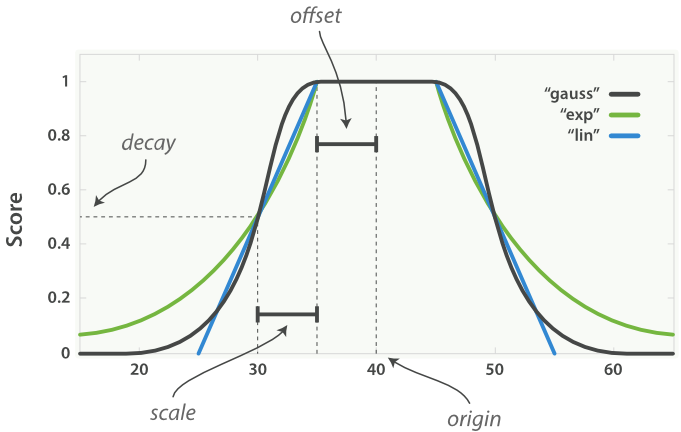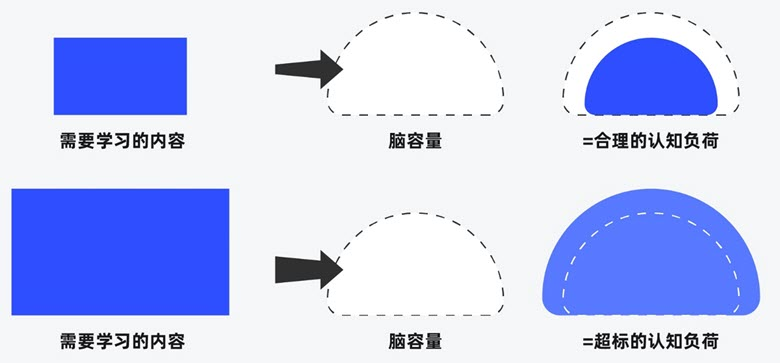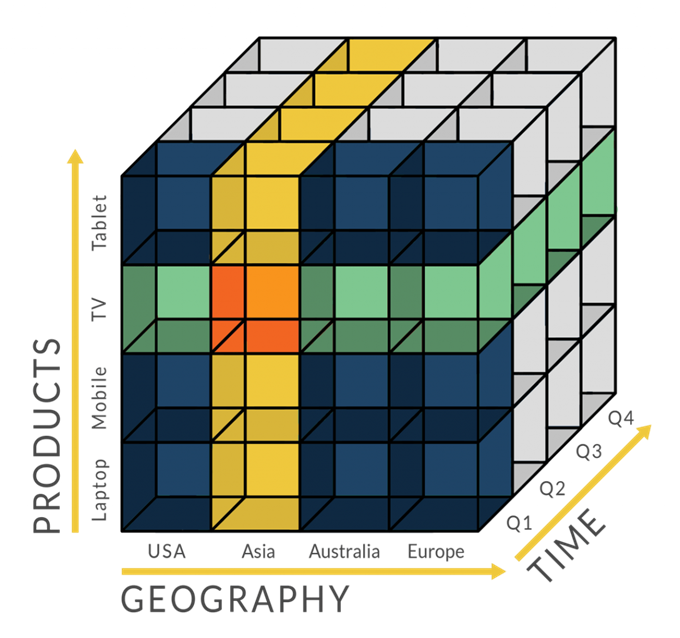# ElasticSearch 实现经纬度附近搜索

ElasticSearch除了支持文本检索外，还支持地理信息检索。它主要支持两种类型的地理查询：一种是地理点(geo_point)，即经纬度查询，另一种是地理形状查询(geo_shape)，即支持点、线、圈、多边形查询等。

## ElasticSearch实现经纬度附近搜索

1、创建映射(mapping)

import requests
import json
attractions_url = "http://localhost:9200/attractions?include_type_name=true"
data = {
"mappings": {
"hotel": {
"properties": {
"name": {
"type": "text"
},
"location": {
"type": "geo_point"
}
}
}
}
}

r = requests.put(url=attractions_url, json=json_data)
print(r.status_code)
print(r.text)

2、添加数据（插入文档）

import requests
import json
import pandas as pd
for index, row in df.iterrows():
url = "http://localhost:9200/attractions/hotel/{0}".format(index)
data = {"name": str(int(row['hotel_id'])), "location": {"lat": row['lat'], "lon": row['lon']}}
r = requests.put(url, json=json_data)

• string：格式为lat,lon
• double []：格式为[lon, lat]
• json：格式为{ “lat”: lat, “lon”: lon}

3、查询

Elasticsearch 的地理位置查询，实际是使用过滤器对所有文档进行过滤。它支持以下四种查询方式：

• geo_bounding_box：查询矩形范围内的点
• geo_distance：查询中心点距离范围内的点
• geo_distance_range：查询中心点最小距离和最大距离之间的点
• geo_polygon：查询多边形范围内的点（不推荐使用）

import requests
import json
url = "http://localhost:9200/attractions/hotel/_search"
lat, lon = 34.514020, 113.191598
data = {
"query": {
"bool": {
"must": {
"match_all": {}
},
"filter": {
"geo_distance": {
"distance": "10km",
"location": {
"lat": lat,
"lon": lon
}
}
}
}
}, "sort": [
{
"_geo_distance": {
"location": [
{
"lat": lat,
"lon": lon
}
],
"unit": "m",
"distance_type": "arc",
"order": "asc",
"validation_method": "STRICT"
}
}
]
}
r = requests.get(url, json=json_data)

• arc：最慢但最精确的是 arc 计算方式，这种方式把世界当作球体来处理。不过这种方式的精度有限，因为这个世界并不是完全的球体。
• plane：plane 计算方式把地球当成是平坦的，这种方式快一些但是精度略逊。在赤道附近的位置精度最好，而靠近两极则变差。
• sloppy_arc：如此命名，是因为它使用了 Lucene的SloppyMath 类。这是一种用精度换取速度的计算方式，它使用 Haversine formula 来计算距离。它比 arc 计算方式快 4 到 5 倍，并且距离精度达9%。这也是默认的计算方式。

## ElasticSearch按距离打分的配置

ElasticSearch除了按照距离排序外，还支持按距离打分并且与其他评分一起应用。

• Origin：中心点 或字段可能的最佳值，落在原点 origin 上的文档评分 _score 为满分0。
• Scale：衰减率，即一个文档从原点 origin 下落时，评分 _score 改变的速度。
• Decay：从原点 origin 衰减到 scale 所得的评分 _score ，默认值为5 。
• Offset：以原点 origin 为中心点，为其设置一个非零的偏移量 offset 覆盖一个范围，而不只是单个原点。在范围 -offset <= origin <= +offset 内的所有评分 _score 都是0 。gauss

$$S(doc) = \exp(-\frac{\max(0,|\text{fieldvalue}_{doc}-\text{origin}|-\text{offset})^2}{2\sigma ^2})$$

$$\sigma ^2=-\frac{\text{scale}^2}{2\cdot \ln(\text{decay})}$$

exp

$$S(doc) = \exp(\lambda \cdot max(0,|\text{fieldvalue}_{doc}-\text{origin}|-\text{offset}))$$

$$\lambda = \frac{\ln(\text{scale})}{\text{scale}}$$

linear

$$S(doc) = \max(\frac{s-\max(0,|\text{fieldvalue}_{doc}-\text{origin}|-\text{offset})}{s},0)$$

$$s = \frac{\text{scale}}{1.0-\text{decay}}$$##### 界面设计和游戏设计中的认知负荷##### 理解认知偏差